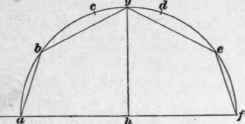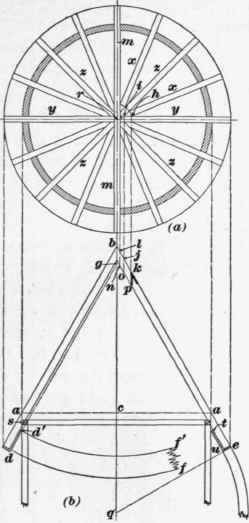The relative pitch of the top and side slopes of a Mansard roof may be determined in several ways, one of which, known as Belidor's method, is shown in Fig. 53.

Upon the center h of a line af, representing the span of the roof, a semicircle acdf is drawn and its circumference divided into five equal parts at a, b, c, d, e,f. By connecting ab and cf the pitch of the sides of the roof is obtained, while on connecting b and e with the ridge or apex at g, the proper slope for the top or summit slope is secured. Variations from this rule are common in practice, but the above proportions are such as will usually give most satisfactory structural results.

142. In Fig. 54 is shown a plan (a) and the section (b) through the lower slope of a Mansard roof, while at (c) is shown the elevation of the angle rib at ae or df in Fig. 52.Fig. 52.Fig. .53.

In the plan (a) uk" x is a corner, or angle, of the building at the lowest portion of the sloping sides of the roof, and is designated by k in the section (b). The line shy, in the plan, is the upper edge of the sloping side, designated in the section (b) by r. If we now project up from the curved side in the section (b) any number of points, such as c, d, r, marking their intersections with the line k" g, at j, i, h, and from these points, and at right angles to k" g, lay off the lines jl', im', hn', equal to cl, dm, and rn in the section (b), we have, at l', m', n', points in the curve of the angle rib which, if connected by a curved line, will give the profile sought.

The distance k" k' on the angle rib (c) is the height of the point k, in the section (b), above the bottom of the main slope at a.Fig. 54.

143. In Fig. 55 is a framing plan (a) and sectional elevation (b) of a conical roof, the height of which is cb and the pitch ba. The rafters a b are notched over the plate in the same manner as though the roof were a plain gable, but the mitering of the upper ends is somewhat different, and can be best understood by reference to the plan (a).

The first pair of rafters m, m have a plumb-cut exactly the same as a gable rafter, and are butted squarely together at r, while the second pair y are similarly cut and butted and spiked at their upper ends against the first pair. The rafters z are then cut the same as y, but with the addition of a cheek-cut at an angle of 45° on both sides of the upper end; and the rafters x are made similar to z, except that the cheek-cuts are each 22 1/2°, instead of 45°.

In the elevation (b) the rafters are shown overhanging the plate from a to e in order to form the eaves, and the length e b will be the length of the two rafters shown in the plan at in; from bc is then measured off, at right angles, the half thickness of the rafter m, and through the point thus located, a perpendicular line is drawn, cutting the rafter at nl; then will el be the length of the rafters shown in the plan at y. The point i, where the edge of the rafter z intersects the edge of the rafter y, if projected down to the elevation (b), will cut the elevation of the rafter at oj, and/r will then be the length to the cheek-cut, of the rafters shown in the plan at z. The length of the rafters x to the cheek-cut is then found by projecting the points of intersection, of adjacent sides of x and y, to the top of the rafter in elevation, cutting the rafter at p k; k e will then be the length of the rafters to the cheek-cut, as shown in the plan at x. The angle e kp will be the plumb-cut for all the rafters in the roof.Fig. 55.

144. The wall plate, shown in the elevation at s, is cut out of two thicknesses of 2-inch plank, each piece being long enough to receive the feet of four rafters. When the plate is built in position, it is laid up in two thicknesses, no two joints of which are to be in line perpendicularly, and the joints on the under side to be well supported by studs.

The under side of the rafters, where they project beyond the plate, is sometimes lined, or sheathed, with a board t u called the plancher, or soffit, and the ends of the rafters at e u are then faced with a board called the facia. The proper curvature of the edges of the plancher d f is found by drawing the line dg parallel to the inside of the rafter, and at a distance from it equal to the thickness of the plancher; this line will intersect the axis b c of the roof at g; then, with g as a center and radii gd and gd', describe the arcs d f and d' f', which will give the curvature required. The length of the plancher on the line df will, of course, be equal to the circumference of the roof at d.

The roofing boards over the rafters are applied in the same manner as is described hereafter, in connection with the covering of domes, illustrated, subsequently, in Art. 205.

145. Where a conical roof intersects the pitch of another roof, it is necessary to resort to particular framing in each case. Fig. 56 shows the plan of a conical tower roof a b c, intersecting a flat single slope efhg, the line of intersection being the curve a dc. In Fig. 57 the plan A of the tower is divided into sixteen equal parts by the radial lines y' a', y' x', y' b', etc., each one of which represents the center line of a rafter. The sectional elevation B shows the apex of the roof at y and the rafters and their slopes at yb and y d, while db is the line of the plate. The rafter vp shows the slope of the main roof to which it belongs, and the point w shows the highest point in the line of intersection between the conical tower and the flat roof.Fig. 56.